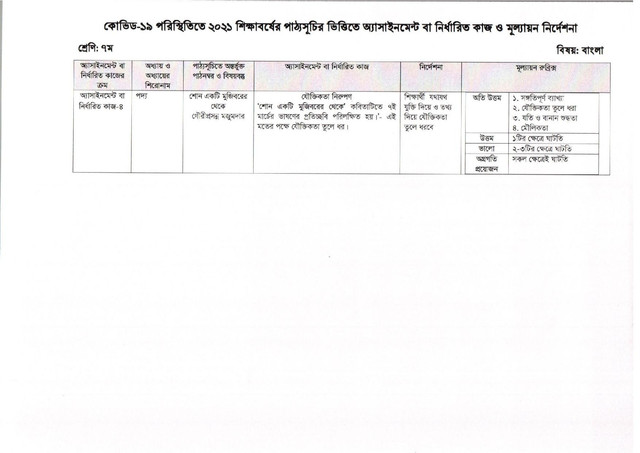Assignment

# Class 7 Assignment 13th week 2022 (Bangla and Math)

The National Curriculum and Textbook Baird (NCTB) has released the 13th week assignment for 6th to 9th class of secondary and higher secondary students of 2022 due to the ongoing Covid-19 overdose. August 25, 2022 The Department of Secondary and Higher Education published the 13th week 2022 assignment of the seventh grade;

The 13th week assignments for seventh grade students prepared by the Department of Secondary and Higher Education and published by the Department of Secondary and Higher Education were given in one page PDF format; [ বাংলায় দেখুন ]

## Class 7 13th Week Bangla AssignmentSubject: Bangla Assignment Number: 04

Assignment and Chapter Title: Chapter-Verse;
Learning Outcomes / Contents: Gauriprasanna Majumdar from Shane A Mujibur;

Assignment:

Rhetoric is reflected in the March 7 speech in the poem “Shane from a Mujibur”; Highlight the rationale for this view;

Instructions (signal / step / circumference):

The student will present the rationale with appropriate reasoning and information;

## Class 7 13th Week Math AssignmentSubject: Mathematics, Assignment Number: 03

Assignment and Chapter Title: Chapter: IV; Multiplication and division of algebraic sums;

Assignment:

2. If A = a2 – a + 1, B = a2 + 0 + 1 and C = a + a2 + 1, show that BC – B2 – A = 0 3. 18 × 3 + 15 × 2 = Enter the first bracket with a (-) sign before the second and third brackets of x – 2 and divide the result by the product of (2x + 1) and (3x + 2);

Learning Outcomes / Content:

1. Multiplication of algebraic numbers;

2. Multiplication and division of algebraic sums;

3. Problems related to addition, subtraction, multiplication and division of algebraic quantities;

1. In case of multiplication of algebraic sum, mention the rule of multiplication and the rule of distribution with examples

Instructions (signal / step / circumference):

1. Students will cite the rules of multiplication with examples to multiply algebraic quantities;

2. Will verify the veracity of the equation by simplifying the sum on the left and setting the values ​​of A, B and C in the correct process;

3. In the correct process, the second and third terms will be enclosed and the amount obtained will be divided by the product of the given amount;

For the convenience of 7th class 2022 students, one page assignment of 13th week was given in the form of PDF;

Download the PDF of your 13th week assignment on one page by clicking on the desired button below;